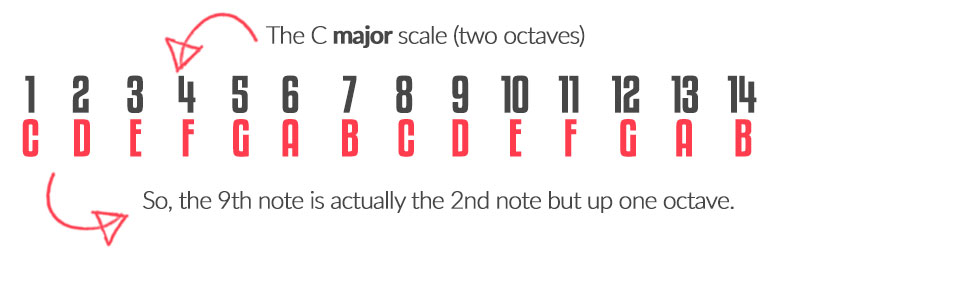9TH CHORDS

To kick off this course we answer the first basic question... 'Where does the 9th come from if the scale only has 7 notes?' As with most theoretical questions, the answer is simple but only if you know it!

## Summary: Finding the 9th

When we are dealing with 9th, 11th or 13th chords we need to extend our basic major scale to two octaves. That is how we find those notes. Have a look at this diagram:As you can see we have extended the scale by a second octave, which are exactly the same notes but all higher. It suddenly because clear therefore that the 9th note is identical to the 2nd note. Calling it a 9th therefore, helps us realise that the note is typically higher in the chord, and needs to include notes from the previous octave as well as the ninth. So, get the two octave C major scale drawn out and we'll go start the first chord in the next lesson.

Ready to move on? Remember to check out every lesson in this unit first – then try the next unit...

Our first 9th chord to study is the major 9th. It always makes sense to start with major as you are never altering the scale, just picking notes from it instead. This makes the scale formulas easy to remember and sets us up for the more difficult formulas.